# Tangent of a circle and the circle circumscribes a triangle: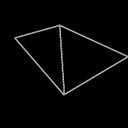(Edited)

Hello math bugs(🐞) and hivers(🐝)
I hope you are strong and stout and doing good in life.

Well come back to another interesting maths problem.

In the figure below you can see a O-centric circle and a tangent PQ at point B on the circle.There is a ∆ABC circumscribed by the circle.Cord AC is given by √3 cm. We need to find out ∠PBC.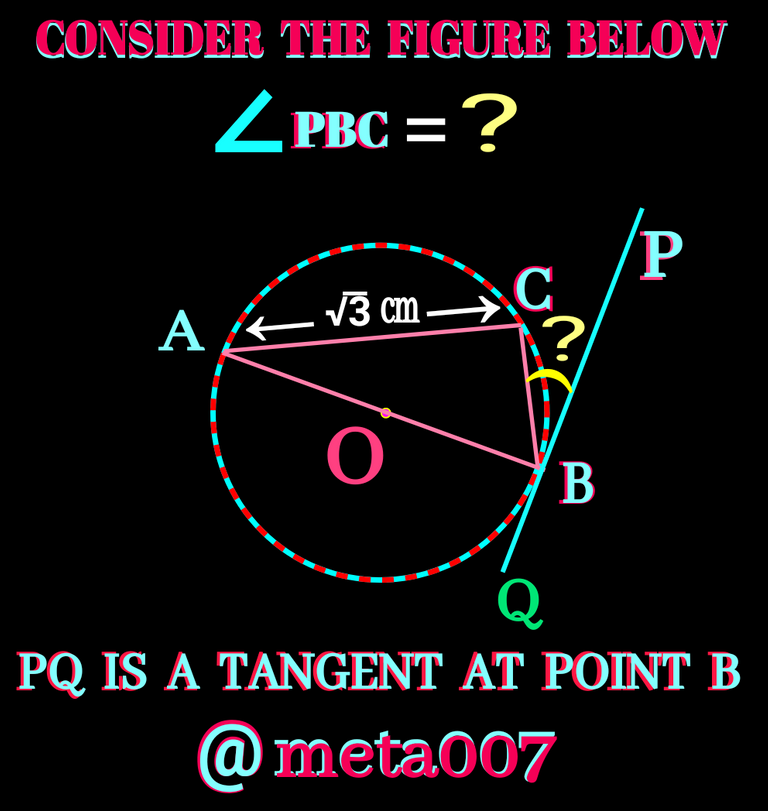Before heading towards solution please give it a try yourself first.let me mention some geometric facts what we need to know to solve it.

1️⃣ The line segment joining centre of the circle and point of contact of the tangent is always perpendicular to the tangent. Check the following figure: OB⊥ PQ and hence ∠QBO = ∠PBO =90°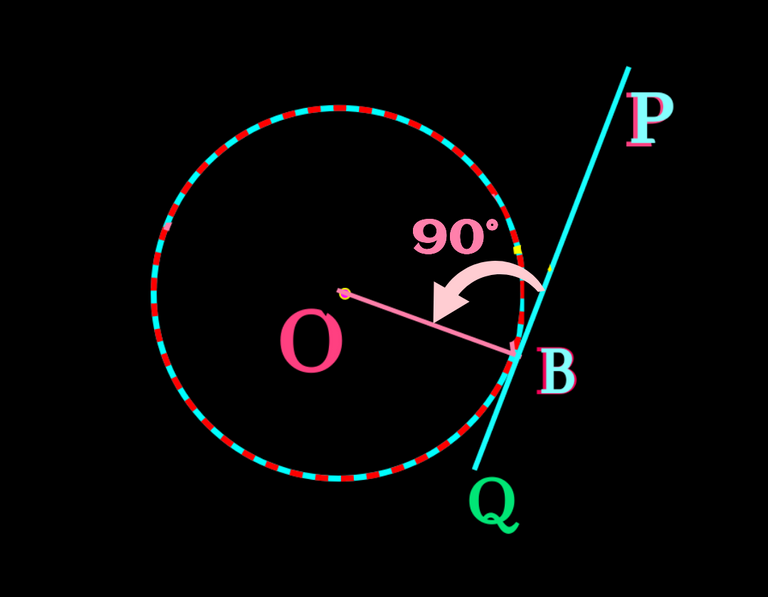2️⃣ Semi-circular angle is always a right angle. Check the figure below: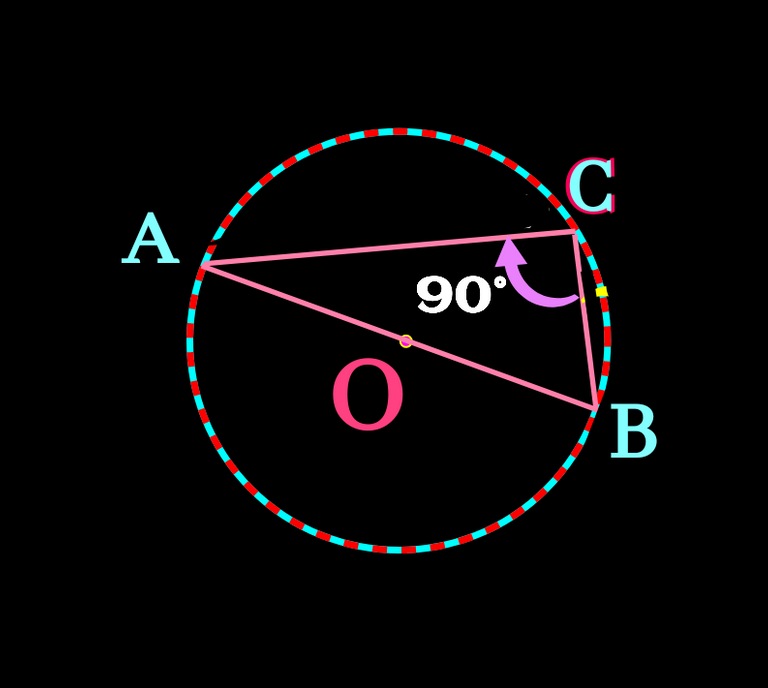3️⃣ Trigonometric ratios: When the angles of a right angle triangles become 30°, 60° and 90°, the ratio of opposite respective sides are 1 : √3 : 2 as shown in the figure below.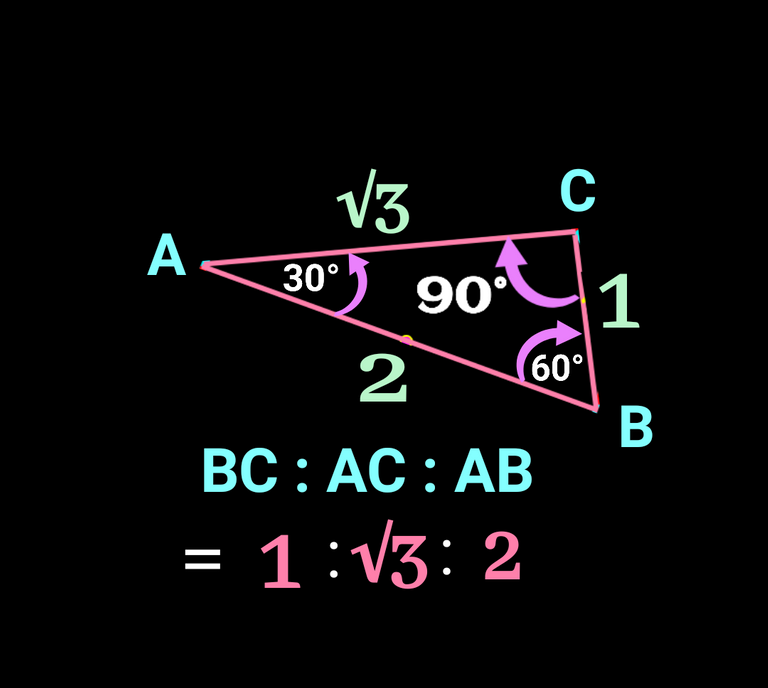With the help of above three consideration we can easily find the required angle.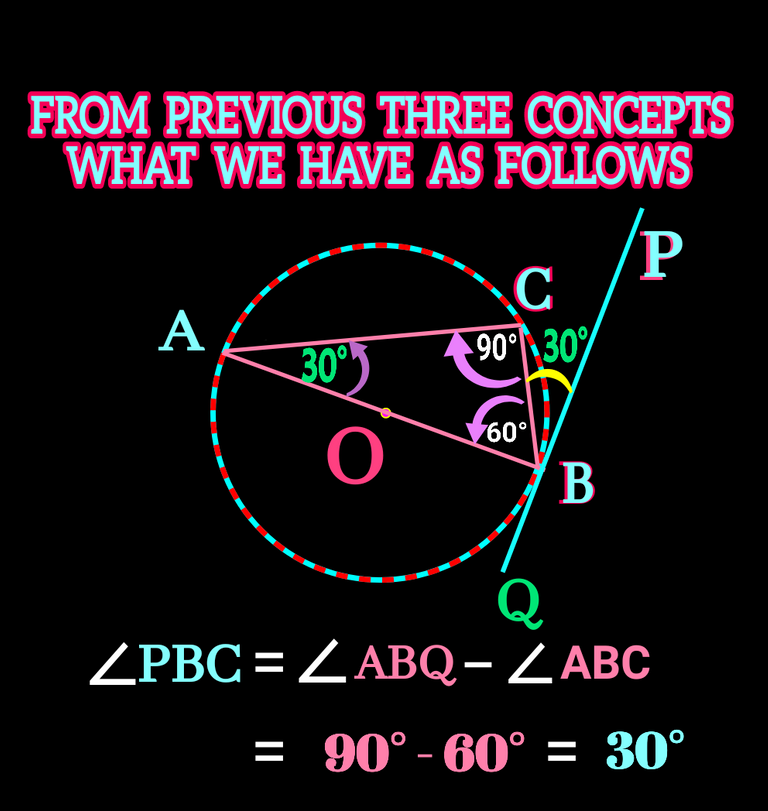✅✅The problem today is a special case of it's kind but the actual concept is not limited to given right angle triangle.The concept is more liberal.Let's get into it:

If a circle circumscribes a triangle, a tangent to the circle at any point of the vertices of the triangle always makes two angles with two sides of the triangle. In the following figure such two angles are ∠PBC and ∠ABQ. Here always ∠BAC =∠PBC and ∠ABQ=∠ACB.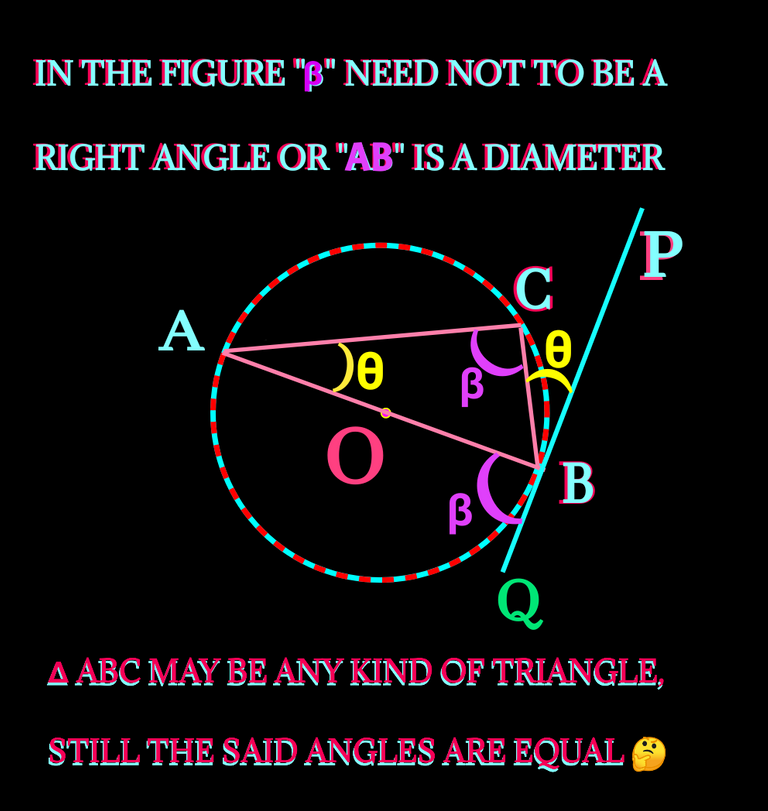LET'S PROVE THE CONCEPT

To prove it let's take another suitable figure for that drawing can easily be understood.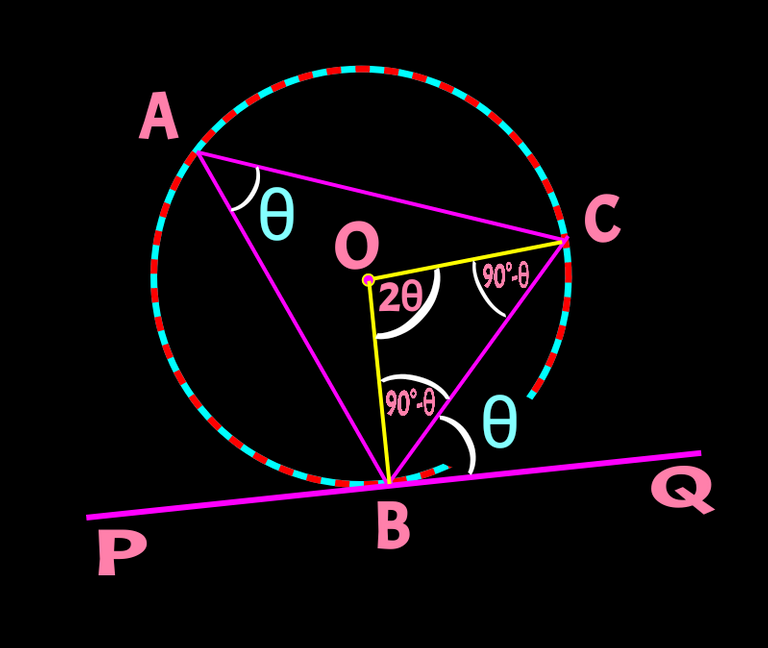let ∠QBC = θ .
So, ∠OBC = (90°-θ) as ∠OBQ = 90°.

As, being radius of same circle BO = CO and hence ∠OBC =∠OCB = (90°-θ)

Now, in ∆OBC, ∠BOC = 180° -(∠OBC+∠OCB)
So ∠BOC = 180° - (90 - θ + 90 - θ)=

We know angle at the centre are twice the angle on the circle( mentioned earlier also).
So, ∠BAC equals to half of ∠BOC.
That means ∠BAC is also equals to θ.

So, ∠QBC = ∠BAC ( Proved)

I hope you have understood the concept(s) very well. Now time for you to solve a related problem. Check this one: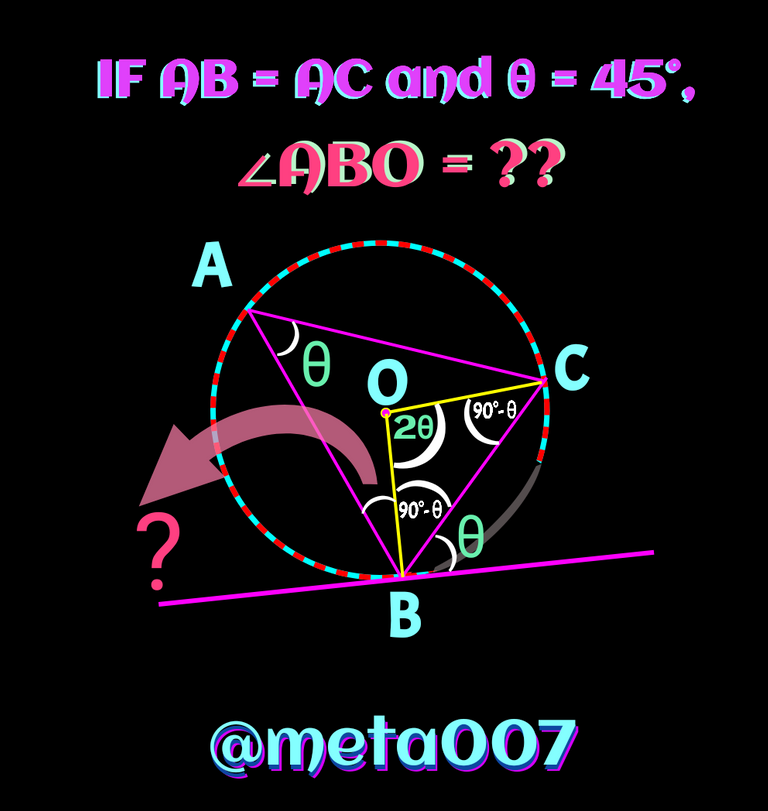I hope you like my hard work needed for framing all the pictures or drawing. Please do share and support it😆.

All the drawing are made by me using Android apps

Please ignore the silly mistakes if any

Thanks you so much for visiting

Have a good day

All is well

Regards: @meta007

0
0
0.000[(180-45)/2]-45 = 22,5 😂🤦‍♂️🙈
Is it true?

0
0
0.000🔥👌
You are awesome. Thank you so much for visiting man.

0
0
0.000You are welcome. 🙏

0
0
0.000Thanks for your contribution to the STEMsocial community. Feel free to join us on discord to get to know the rest of us!

Please consider delegating to the @stemsocial account (85% of the curation rewards are returned).

Thanks for including @stemsocial as a beneficiary, which gives you stronger support.

0
0
0.000